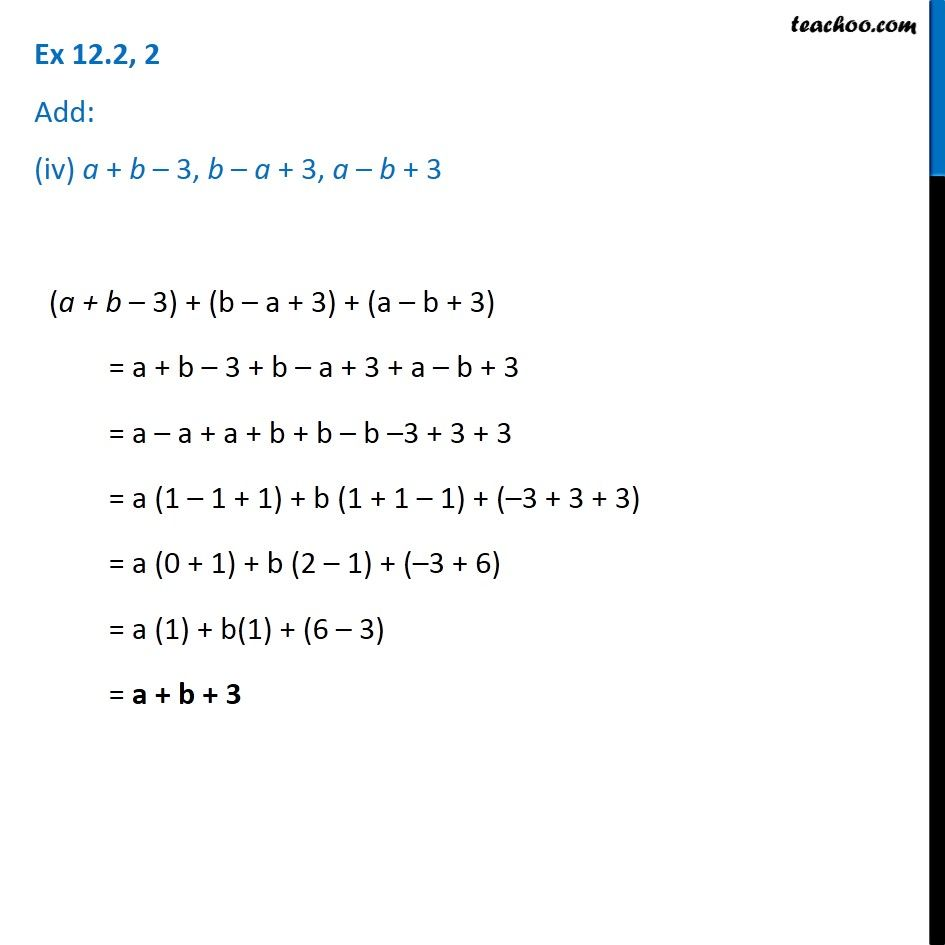Adding and Subtracting Like terms - Worksheet

Chapter 10 Class 7 Algebraic Expressions
Serial order wiseLearn in your speed, with individual attention - Teachoo Maths 1-on-1 Class

### Transcript

Question 2 Add: (iv) a + b – 3, b – a + 3, a – b + 3 (a + b – 3) + (b – a + 3) + (a – b + 3) = a + b – 3 + b – a + 3 + a – b + 3 = a – a + a + b + b – b –3 + 3 + 3 = a (1 – 1 + 1) + b (1 + 1 – 1) + (–3 + 3 + 3) = a (0 + 1) + b (2 – 1) + (–3 + 6) = a (1) + b(1) + (6 – 3) = a + b + 3